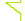Sclub交友聊天~加入聊天室當版主### var day=""; var month=""; var ampm=""; var ampmhour=""; var myweekday=""; var year=""; mydate=new Date(); myweekday=mydate.getDay(); mymonth=mydate.getMonth()+1; myday= mydate.getDate(); myyear= mydate.getYear(); year=(myyear > 200) ? myyear : 1900 + myyear; if(myweekday == 0) weekday=" 星期日 "; else if(myweekday == 1) weekday=" 星期一 "; else if(myweekday == 2) weekday=" 星期二 "; else if(myweekday == 3) weekday=" 星期三 "; else if(myweekday == 4) weekday=" 星期四 "; else if(myweekday == 5) weekday=" 星期五 "; else if(myweekday == 6) weekday=" 星期六 "; document.write(year+"年"+mymonth+"月"+myday+"日 "+weekday);

 / 1 页 返回列表 发帖